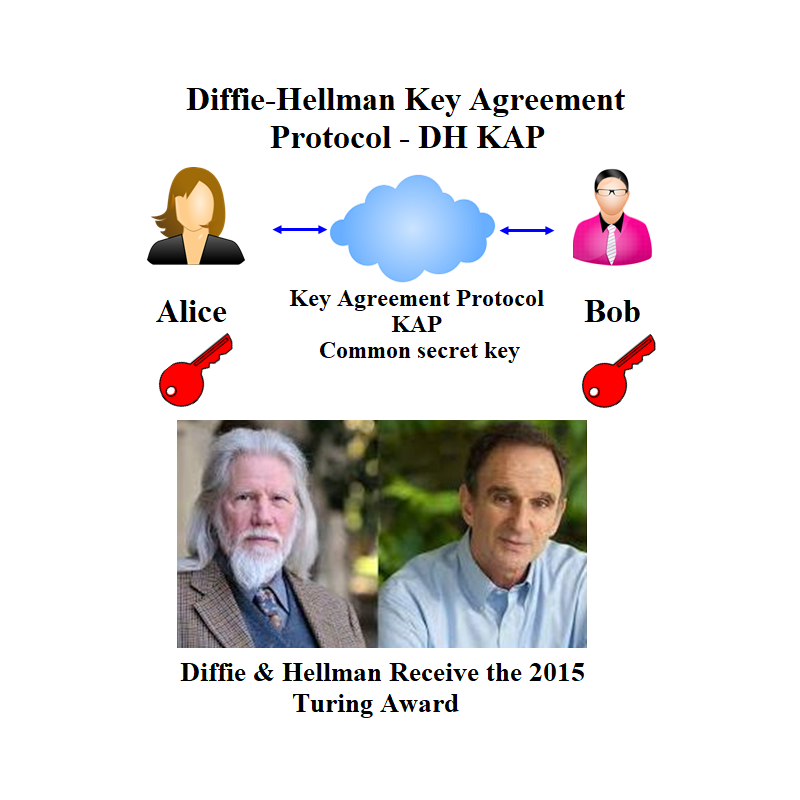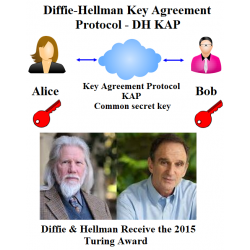•# Diffie-Hellman Key Agreement Protocol (DH-KAP)

€2.00
Tax included

Diffie-Hellman (DH) Key Agreement Protocol (KAP) between two parties: Alice and Bob.

Quantity

This protocol is executed between you named as student Alice and Mentor Bob.

It is strongly recommended that you use Octave software implemented in your local computer rather to use Octave in the server.

Computed numerical parameter values sent by Alice must be included in the input field denoted by brackets [ ]. E.g., if Alice under the Mentor request computed parameters p=201623207 and g=22, then symbolically in the input field she must enter [p,q] which corresponds to enter 201623207, 22 in the input field without brackets.

The following functions are used in the protocol:

>> genstrongprime(bl)     % bl=28 - is recommended bit length of generated strong prime

>> mod_exp(g,x,p);          % function computes modular exponent value gx mod p

>> randi(i);                        % i=26 – is recommended an upper range of generated random integer number

>> dec2bin(decvar);         % decvar – is a decimal variable

>> bin2dec(binvar);         % binvar – is a binary variable

>> binaryxor(binvar1,binvar2);              % function returns bitwise xor between binvar1 and binvar2

3142 Items